Скачать презентацию Statistics for Managers Using Microsoft Excel 5 th

30f2bf343aaf928729d58726b145fca3.ppt

• Количество слайдов: 51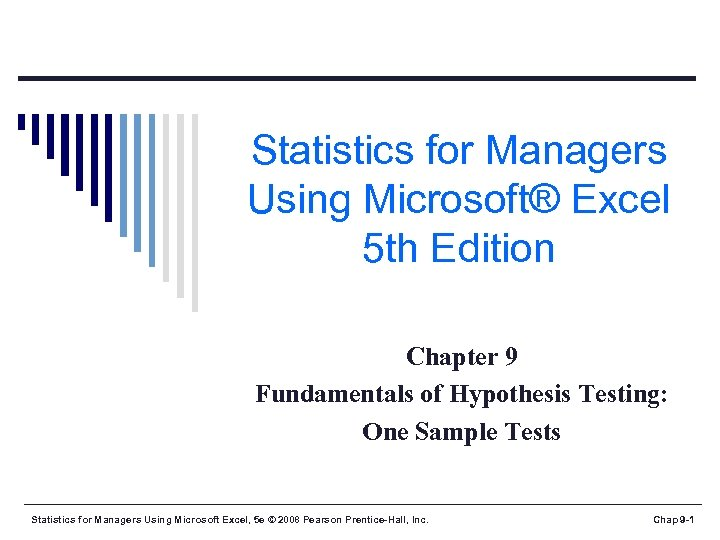Statistics for Managers Using Microsoft® Excel 5 th Edition Chapter 9 Fundamentals of Hypothesis Testing: One Sample Tests Statistics for Managers Using Microsoft Excel, 5 e © 2008 Pearson Prentice-Hall, Inc. Chap 9 -1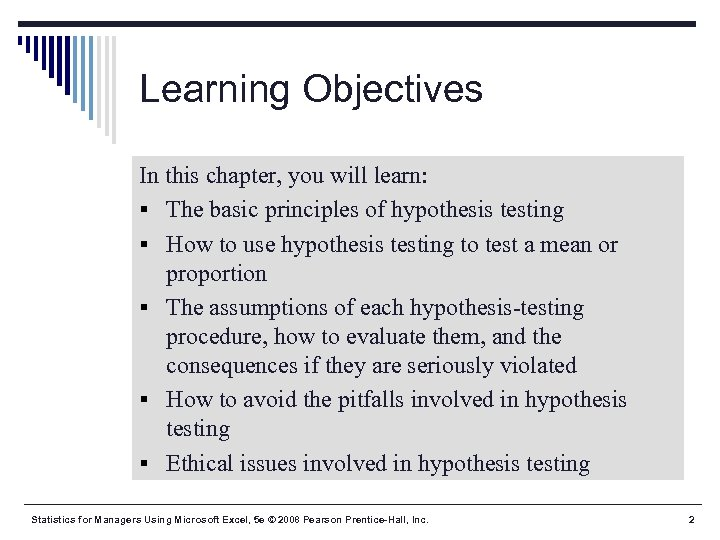Learning Objectives In this chapter, you will learn: § The basic principles of hypothesis testing § How to use hypothesis testing to test a mean or proportion § The assumptions of each hypothesis-testing procedure, how to evaluate them, and the consequences if they are seriously violated § How to avoid the pitfalls involved in hypothesis testing § Ethical issues involved in hypothesis testing Statistics for Managers Using Microsoft Excel, 5 e © 2008 Pearson Prentice-Hall, Inc. 2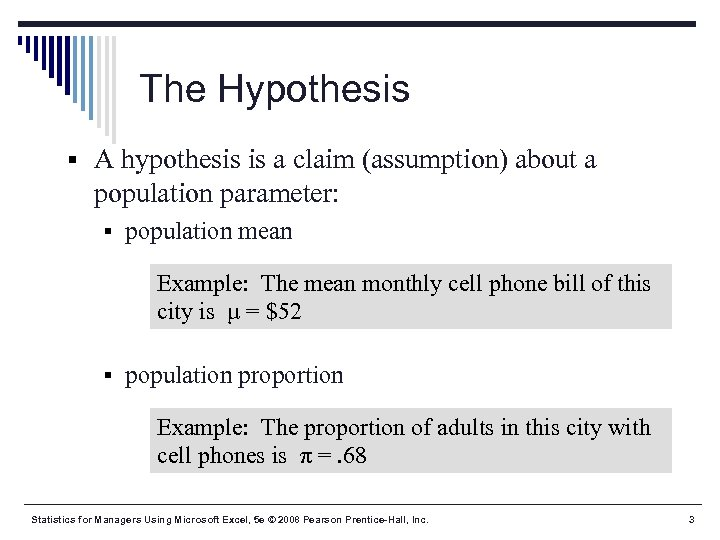The Hypothesis § A hypothesis is a claim (assumption) about a population parameter: § population mean Example: The mean monthly cell phone bill of this city is μ = \$52 § population proportion Example: The proportion of adults in this city with cell phones is π =. 68 Statistics for Managers Using Microsoft Excel, 5 e © 2008 Pearson Prentice-Hall, Inc. 3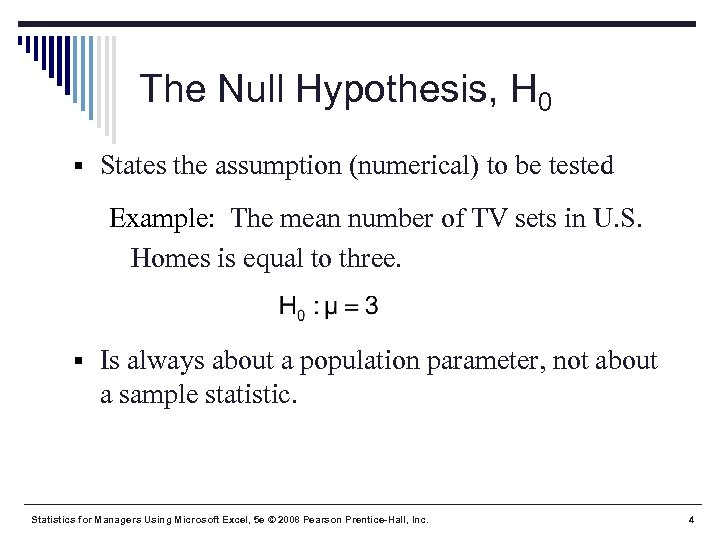The Null Hypothesis, H 0 § States the assumption (numerical) to be tested Example: The mean number of TV sets in U. S. Homes is equal to three. § Is always about a population parameter, not about a sample statistic. Statistics for Managers Using Microsoft Excel, 5 e © 2008 Pearson Prentice-Hall, Inc. 4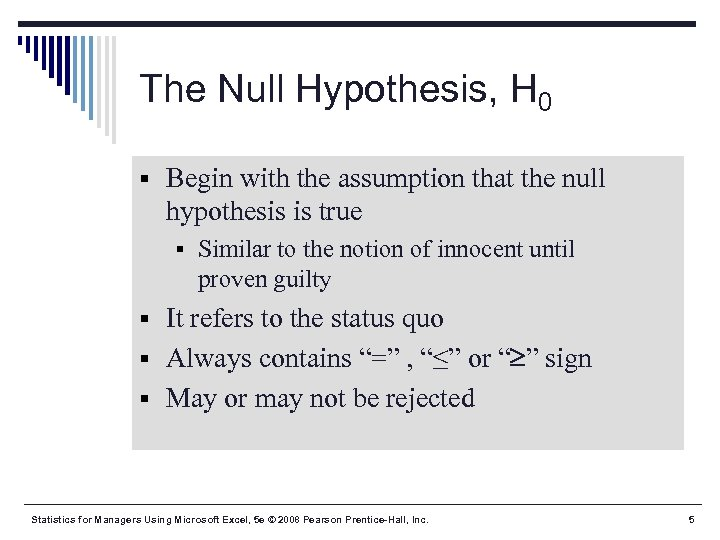The Null Hypothesis, H 0 § Begin with the assumption that the null hypothesis is true § Similar to the notion of innocent until proven guilty § It refers to the status quo § Always contains “=” , “≤” or “ ” sign § May or may not be rejected Statistics for Managers Using Microsoft Excel, 5 e © 2008 Pearson Prentice-Hall, Inc. 5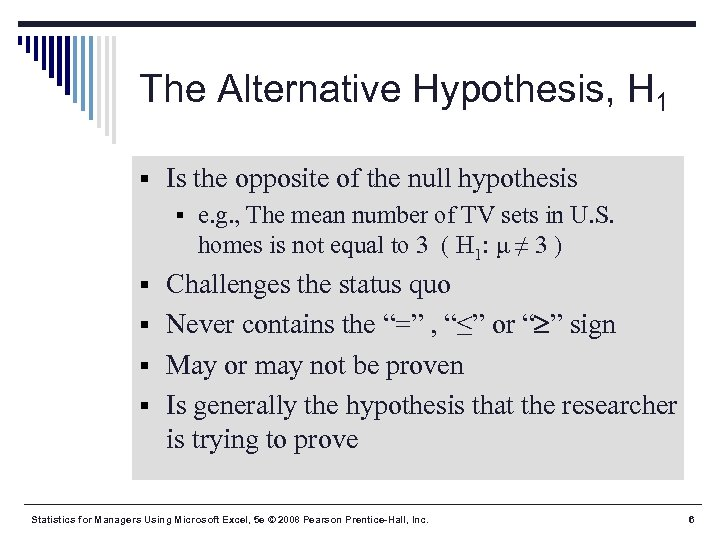The Alternative Hypothesis, H 1 § Is the opposite of the null hypothesis § e. g. , The mean number of TV sets in U. S. homes is not equal to 3 ( H 1: μ ≠ 3 ) § Challenges the status quo § Never contains the “=” , “≤” or “ ” sign § May or may not be proven § Is generally the hypothesis that the researcher is trying to prove Statistics for Managers Using Microsoft Excel, 5 e © 2008 Pearson Prentice-Hall, Inc. 6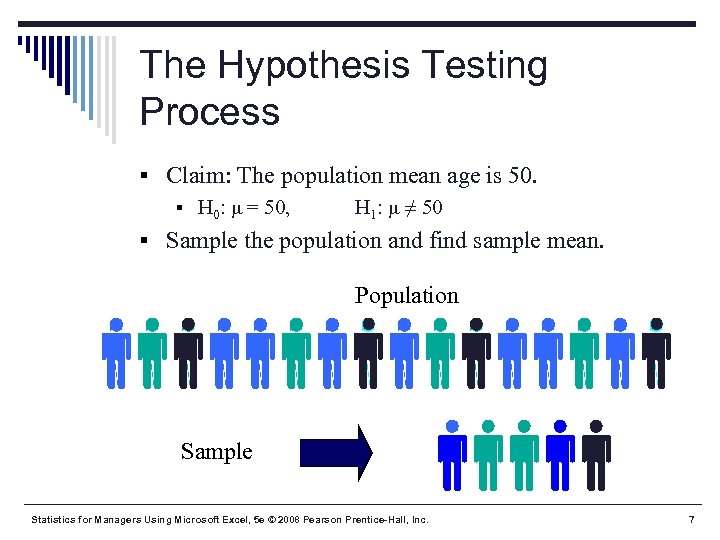The Hypothesis Testing Process § Claim: The population mean age is 50. § H 0: μ = 50, H 1: μ ≠ 50 § Sample the population and find sample mean. Population Sample Statistics for Managers Using Microsoft Excel, 5 e © 2008 Pearson Prentice-Hall, Inc. 7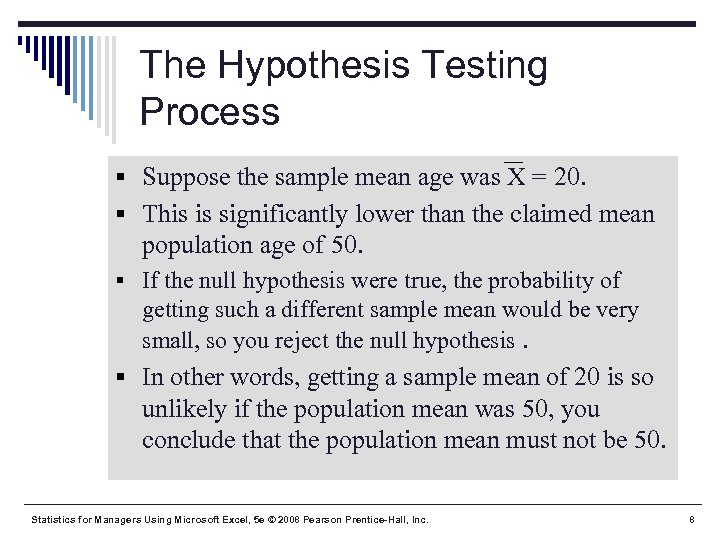The Hypothesis Testing Process § Suppose the sample mean age was X = 20. § This is significantly lower than the claimed mean population age of 50. § If the null hypothesis were true, the probability of getting such a different sample mean would be very small, so you reject the null hypothesis. § In other words, getting a sample mean of 20 is so unlikely if the population mean was 50, you conclude that the population mean must not be 50. Statistics for Managers Using Microsoft Excel, 5 e © 2008 Pearson Prentice-Hall, Inc. 8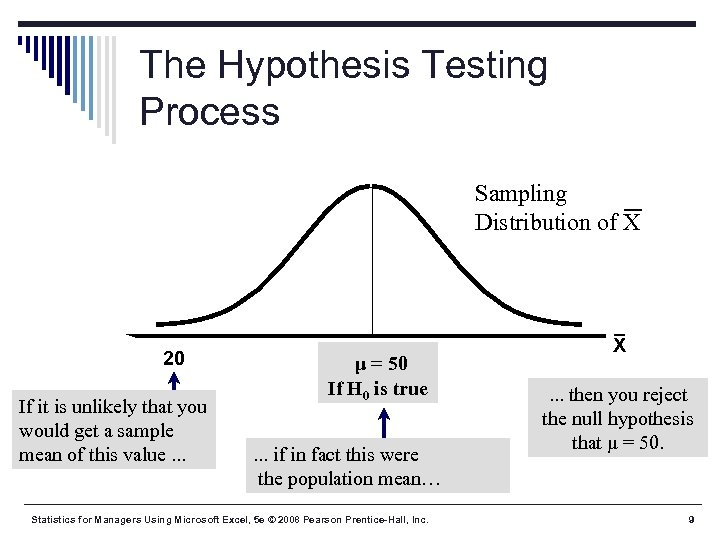The Hypothesis Testing Process Sampling Distribution of X 20 If it is unlikely that you would get a sample mean of this value. . . μ = 50 If H 0 is true. . . if in fact this were the population mean… Statistics for Managers Using Microsoft Excel, 5 e © 2008 Pearson Prentice-Hall, Inc. X. . . then you reject the null hypothesis that μ = 50. 9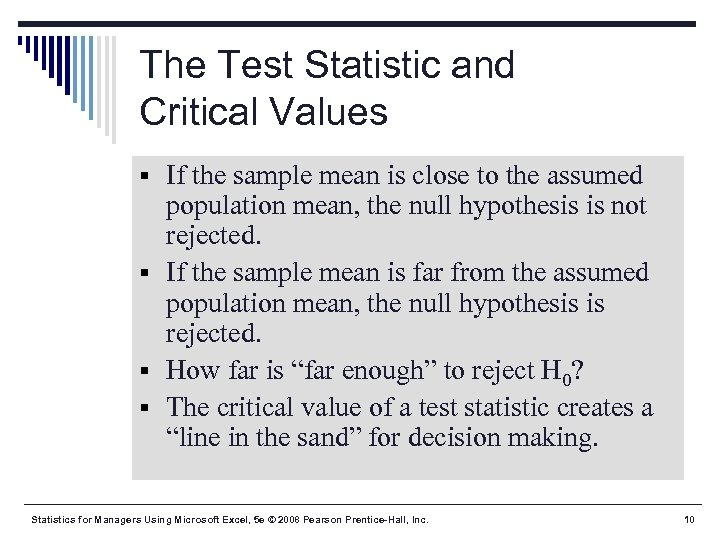The Test Statistic and Critical Values § If the sample mean is close to the assumed population mean, the null hypothesis is not rejected. § If the sample mean is far from the assumed population mean, the null hypothesis is rejected. § How far is “far enough” to reject H 0? § The critical value of a test statistic creates a “line in the sand” for decision making. Statistics for Managers Using Microsoft Excel, 5 e © 2008 Pearson Prentice-Hall, Inc. 10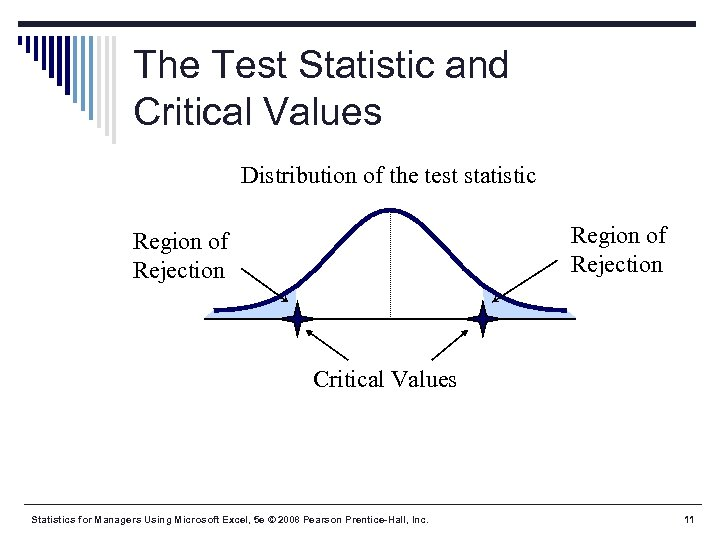The Test Statistic and Critical Values Distribution of the test statistic Region of Rejection Critical Values Statistics for Managers Using Microsoft Excel, 5 e © 2008 Pearson Prentice-Hall, Inc. 11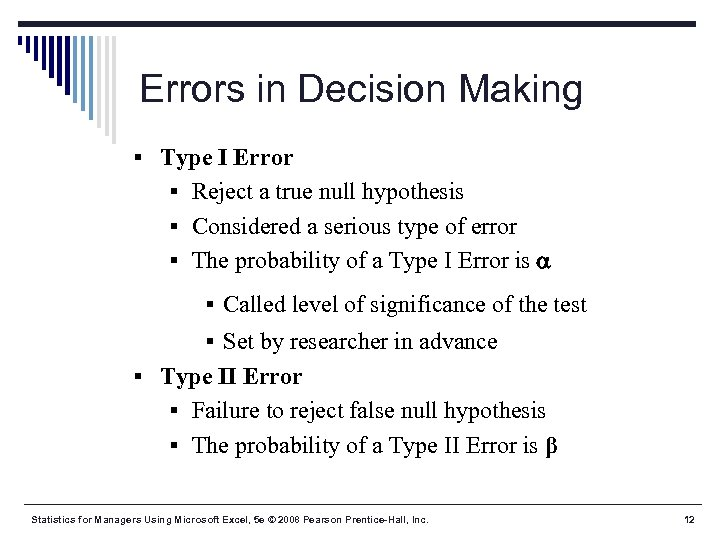Errors in Decision Making § Type I Error § Reject a true null hypothesis § Considered a serious type of error § The probability of a Type I Error is § Called level of significance of the test § Set by researcher in advance § Type II Error § Failure to reject false null hypothesis § The probability of a Type II Error is β Statistics for Managers Using Microsoft Excel, 5 e © 2008 Pearson Prentice-Hall, Inc. 12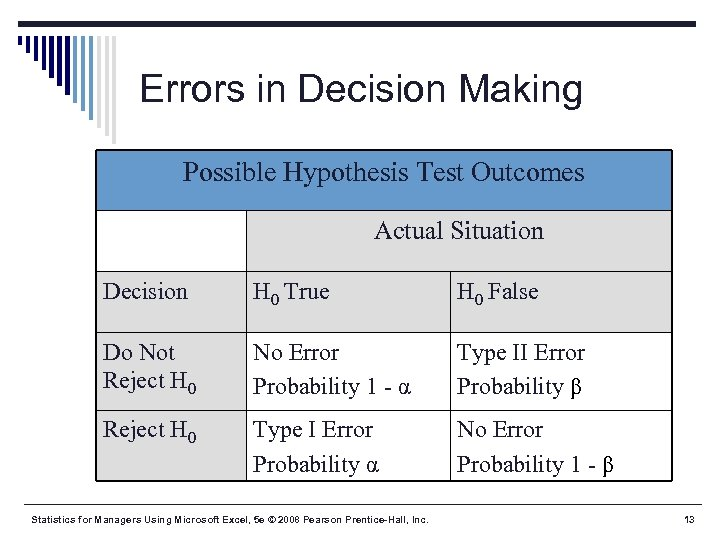Errors in Decision Making Possible Hypothesis Test Outcomes Actual Situation Decision H 0 True H 0 False Do Not Reject H 0 No Error Probability 1 - α Type II Error Probability β Reject H 0 Type I Error Probability α No Error Probability 1 - β Statistics for Managers Using Microsoft Excel, 5 e © 2008 Pearson Prentice-Hall, Inc. 13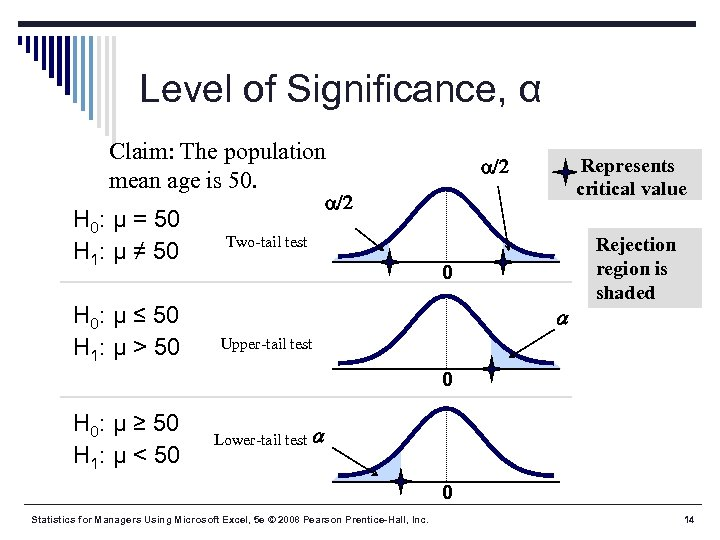Level of Significance, α Claim: The population mean age is 50. H 0: μ = 50 H 1: μ ≠ 50 H 0: μ ≤ 50 H 1: μ > 50 /2 Represents critical value /2 Two-tail test 0 Rejection region is shaded Upper-tail test 0 H 0: μ ≥ 50 H 1: μ < 50 Lower-tail test 0 Statistics for Managers Using Microsoft Excel, 5 e © 2008 Pearson Prentice-Hall, Inc. 14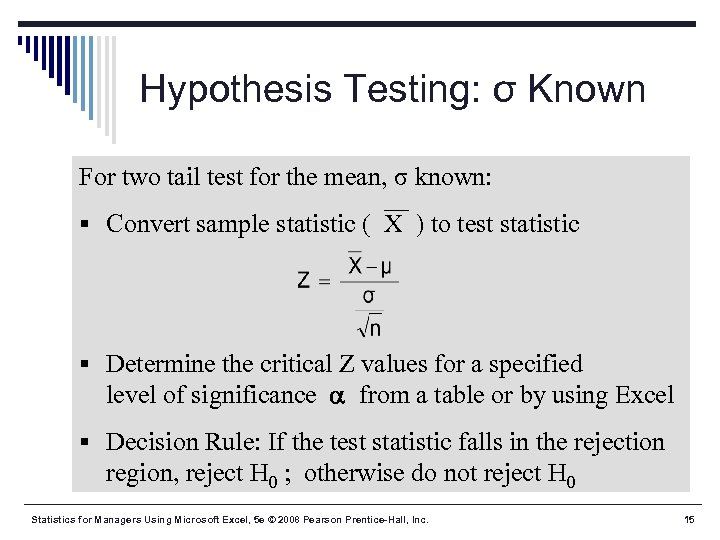Hypothesis Testing: σ Known For two tail test for the mean, σ known: § Convert sample statistic ( X ) to test statistic § Determine the critical Z values for a specified level of significance from a table or by using Excel § Decision Rule: If the test statistic falls in the rejection region, reject H 0 ; otherwise do not reject H 0 Statistics for Managers Using Microsoft Excel, 5 e © 2008 Pearson Prentice-Hall, Inc. 15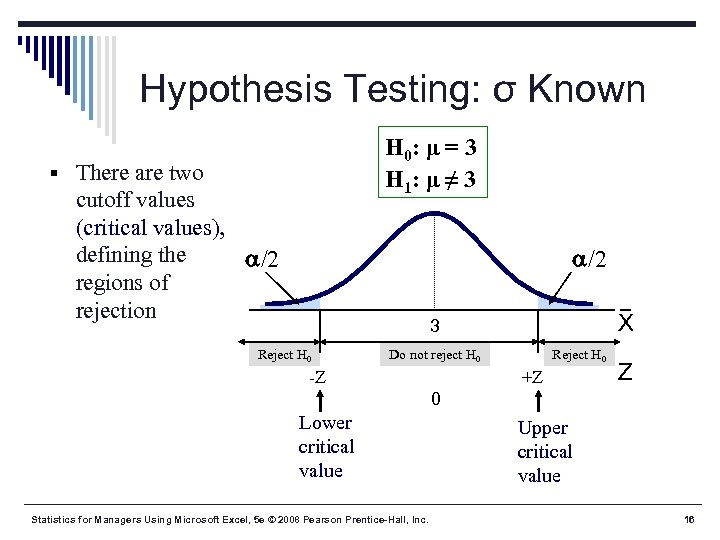Hypothesis Testing: σ Known H 0: μ = 3 H 1: μ ≠ 3 § There are two cutoff values (critical values), defining the /2 regions of rejection /2 X 3 Reject H 0 Do not reject H 0 -Z Reject H 0 +Z Z 0 Lower critical value Statistics for Managers Using Microsoft Excel, 5 e © 2008 Pearson Prentice-Hall, Inc. Upper critical value 16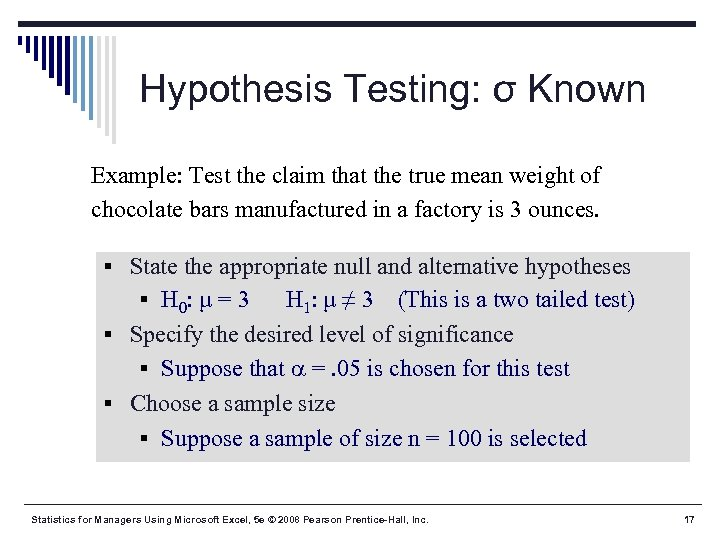Hypothesis Testing: σ Known Example: Test the claim that the true mean weight of chocolate bars manufactured in a factory is 3 ounces. § State the appropriate null and alternative hypotheses § H 0: μ = 3 H 1: μ ≠ 3 (This is a two tailed test) § Specify the desired level of significance § Suppose that =. 05 is chosen for this test § Choose a sample size § Suppose a sample of size n = 100 is selected Statistics for Managers Using Microsoft Excel, 5 e © 2008 Pearson Prentice-Hall, Inc. 17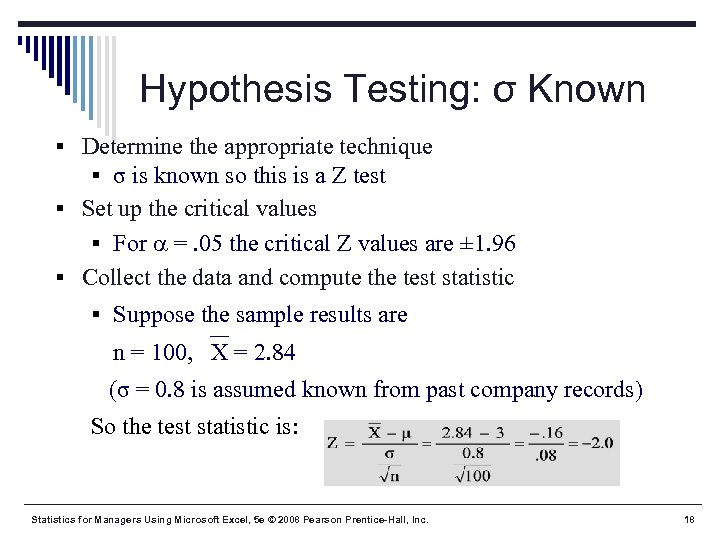Hypothesis Testing: σ Known § Determine the appropriate technique § σ is known so this is a Z test § Set up the critical values § For =. 05 the critical Z values are ± 1. 96 § Collect the data and compute the test statistic § Suppose the sample results are n = 100, X = 2. 84 (σ = 0. 8 is assumed known from past company records) So the test statistic is: Statistics for Managers Using Microsoft Excel, 5 e © 2008 Pearson Prentice-Hall, Inc. 18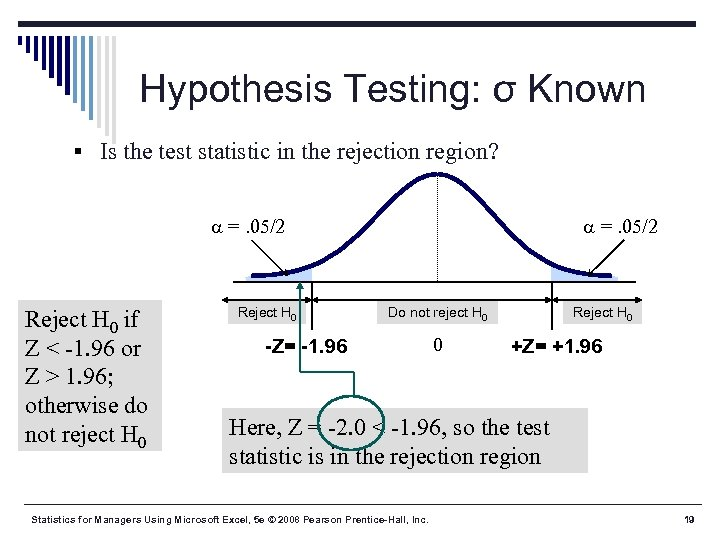Hypothesis Testing: σ Known § Is the test statistic in the rejection region? =. 05/2 Reject H 0 if Z < -1. 96 or Z > 1. 96; otherwise do not reject H 0 Reject H 0 =. 05/2 Do not reject H 0 -Z= -1. 96 0 Reject H 0 +Z= +1. 96 Here, Z = -2. 0 < -1. 96, so the test statistic is in the rejection region Statistics for Managers Using Microsoft Excel, 5 e © 2008 Pearson Prentice-Hall, Inc. 19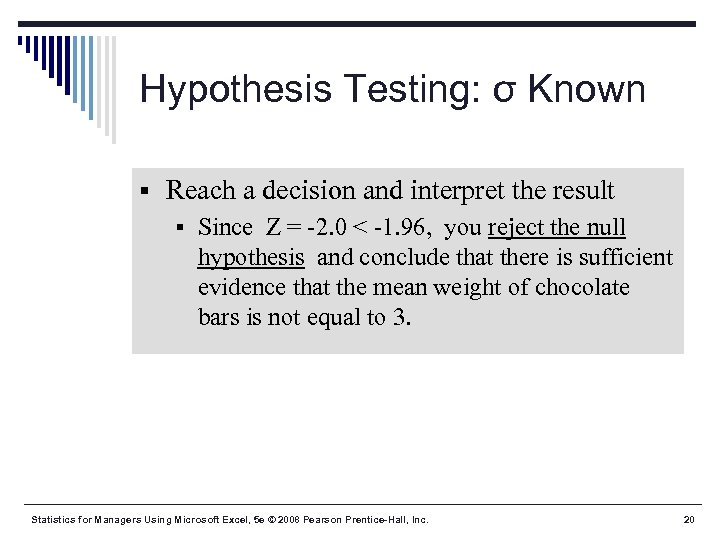Hypothesis Testing: σ Known § Reach a decision and interpret the result § Since Z = -2. 0 < -1. 96, you reject the null hypothesis and conclude that there is sufficient evidence that the mean weight of chocolate bars is not equal to 3. Statistics for Managers Using Microsoft Excel, 5 e © 2008 Pearson Prentice-Hall, Inc. 20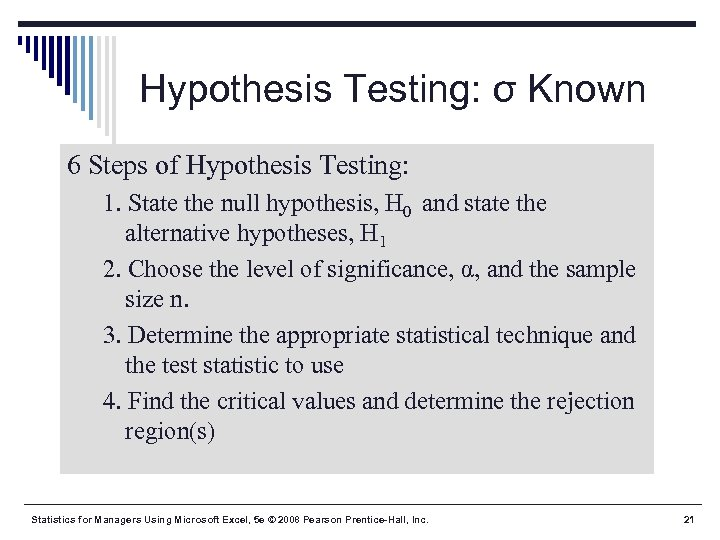Hypothesis Testing: σ Known 6 Steps of Hypothesis Testing: 1. State the null hypothesis, H 0 and state the alternative hypotheses, H 1 2. Choose the level of significance, α, and the sample size n. 3. Determine the appropriate statistical technique and the test statistic to use 4. Find the critical values and determine the rejection region(s) Statistics for Managers Using Microsoft Excel, 5 e © 2008 Pearson Prentice-Hall, Inc. 21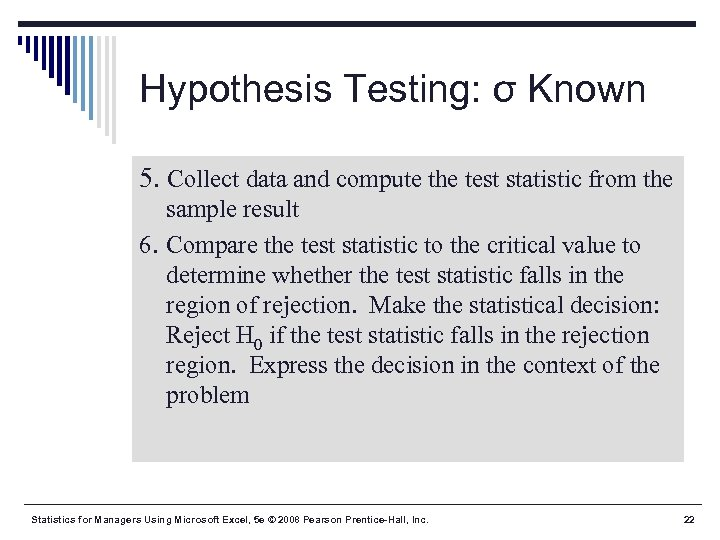Hypothesis Testing: σ Known 5. Collect data and compute the test statistic from the sample result 6. Compare the test statistic to the critical value to determine whether the test statistic falls in the region of rejection. Make the statistical decision: Reject H 0 if the test statistic falls in the rejection region. Express the decision in the context of the problem Statistics for Managers Using Microsoft Excel, 5 e © 2008 Pearson Prentice-Hall, Inc. 22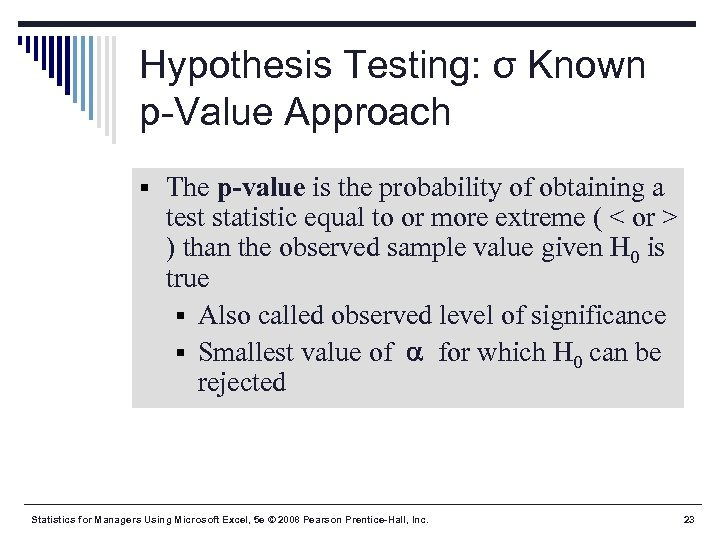Hypothesis Testing: σ Known p-Value Approach § The p-value is the probability of obtaining a test statistic equal to or more extreme ( < or > ) than the observed sample value given H 0 is true § Also called observed level of significance § Smallest value of for which H 0 can be rejected Statistics for Managers Using Microsoft Excel, 5 e © 2008 Pearson Prentice-Hall, Inc. 23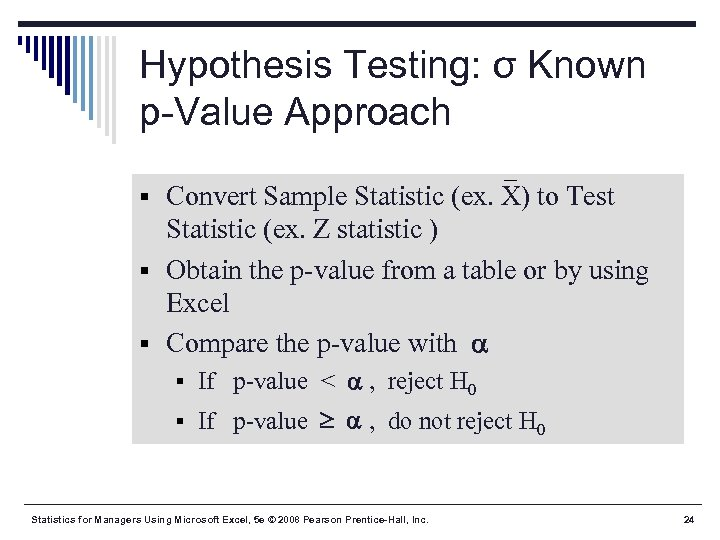Hypothesis Testing: σ Known p-Value Approach § Convert Sample Statistic (ex. X) to Test Statistic (ex. Z statistic ) § Obtain the p-value from a table or by using Excel § Compare the p-value with § If p-value < , reject H 0 § If p-value , do not reject H 0 Statistics for Managers Using Microsoft Excel, 5 e © 2008 Pearson Prentice-Hall, Inc. 24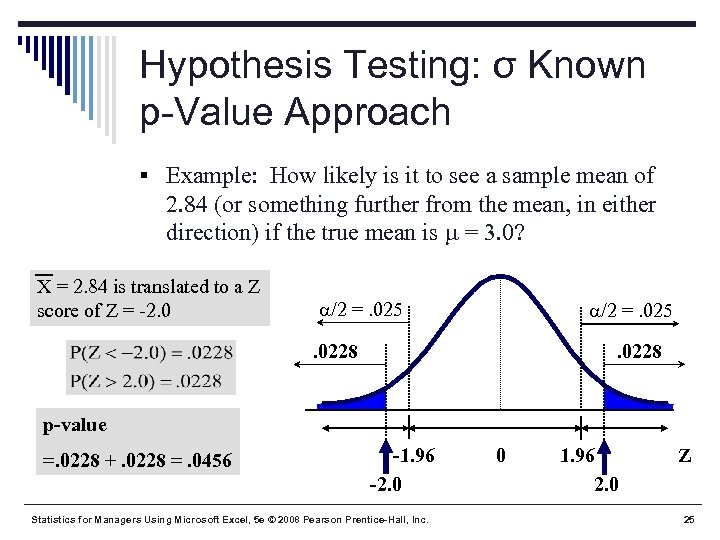Hypothesis Testing: σ Known p-Value Approach § Example: How likely is it to see a sample mean of 2. 84 (or something further from the mean, in either direction) if the true mean is = 3. 0? X = 2. 84 is translated to a Z score of Z = -2. 0 /2 =. 025 . 0228 p-value =. 0228 +. 0228 =. 0456 -1. 96 -2. 0 Statistics for Managers Using Microsoft Excel, 5 e © 2008 Pearson Prentice-Hall, Inc. 0 1. 96 2. 0 Z 25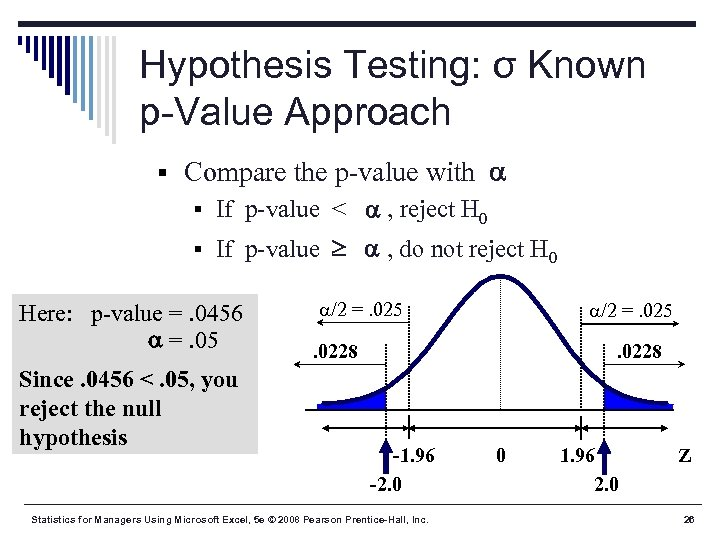Hypothesis Testing: σ Known p-Value Approach § Compare the p-value with § If p-value < , reject H 0 § If p-value , do not reject H 0 Here: p-value =. 0456 =. 05 Since. 0456 <. 05, you reject the null hypothesis /2 =. 025 . 0228 -1. 96 -2. 0 Statistics for Managers Using Microsoft Excel, 5 e © 2008 Pearson Prentice-Hall, Inc. 0 1. 96 2. 0 Z 26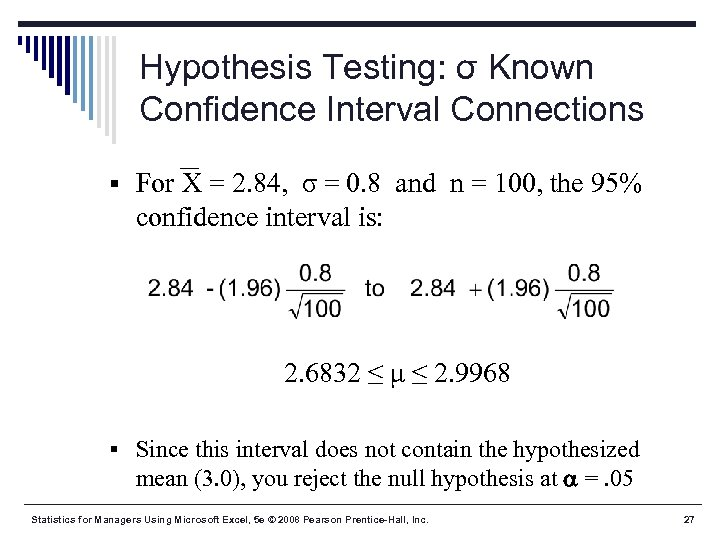Hypothesis Testing: σ Known Confidence Interval Connections § For X = 2. 84, σ = 0. 8 and n = 100, the 95% confidence interval is: 2. 6832 ≤ μ ≤ 2. 9968 § Since this interval does not contain the hypothesized mean (3. 0), you reject the null hypothesis at =. 05 Statistics for Managers Using Microsoft Excel, 5 e © 2008 Pearson Prentice-Hall, Inc. 27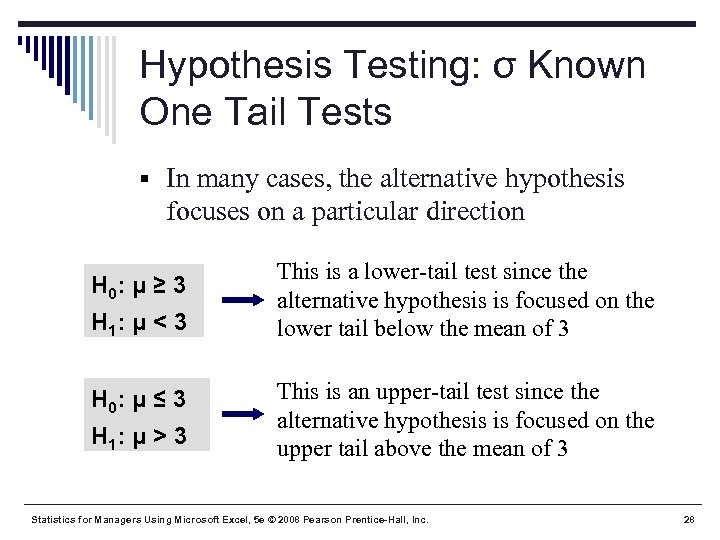Hypothesis Testing: σ Known One Tail Tests § In many cases, the alternative hypothesis focuses on a particular direction H 0: μ ≥ 3 H 1: μ < 3 H 0: μ ≤ 3 H 1: μ > 3 This is a lower-tail test since the alternative hypothesis is focused on the lower tail below the mean of 3 This is an upper-tail test since the alternative hypothesis is focused on the upper tail above the mean of 3 Statistics for Managers Using Microsoft Excel, 5 e © 2008 Pearson Prentice-Hall, Inc. 28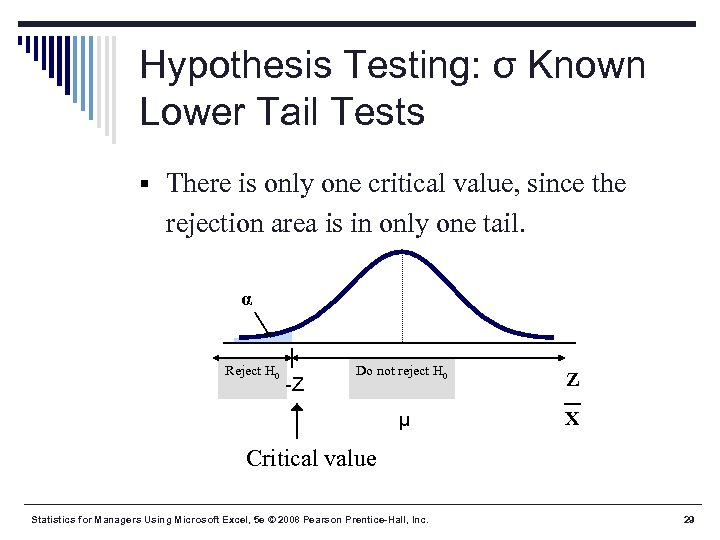Hypothesis Testing: σ Known Lower Tail Tests § There is only one critical value, since the rejection area is in only one tail. α Reject H 0 Z μ -Z Do not reject H 0 X Critical value Statistics for Managers Using Microsoft Excel, 5 e © 2008 Pearson Prentice-Hall, Inc. 29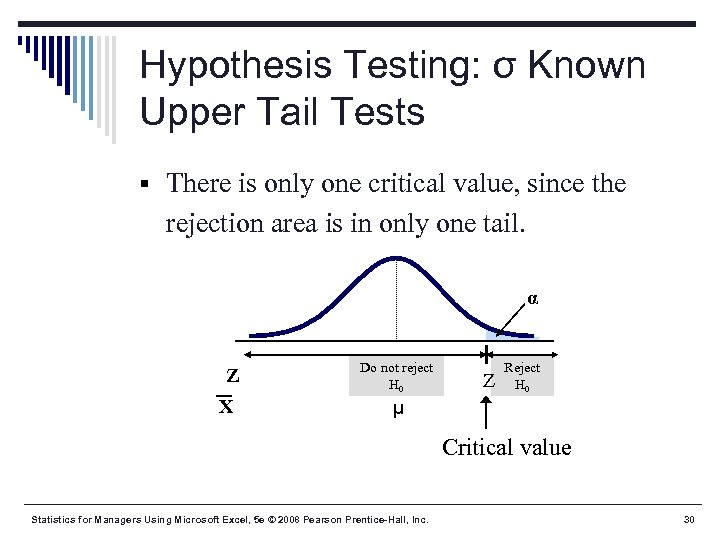Hypothesis Testing: σ Known Upper Tail Tests § There is only one critical value, since the rejection area is in only one tail. α Z X Do not reject H 0 Z Reject H 0 μ Critical value Statistics for Managers Using Microsoft Excel, 5 e © 2008 Pearson Prentice-Hall, Inc. 30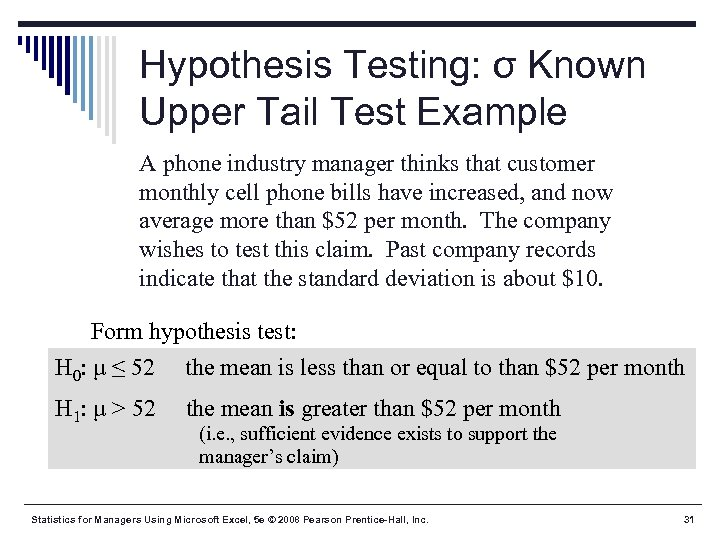Hypothesis Testing: σ Known Upper Tail Test Example A phone industry manager thinks that customer monthly cell phone bills have increased, and now average more than \$52 per month. The company wishes to test this claim. Past company records indicate that the standard deviation is about \$10. Form hypothesis test: H 0: μ ≤ 52 the mean is less than or equal to than \$52 per month H 1: μ > 52 the mean is greater than \$52 per month (i. e. , sufficient evidence exists to support the manager’s claim) Statistics for Managers Using Microsoft Excel, 5 e © 2008 Pearson Prentice-Hall, Inc. 31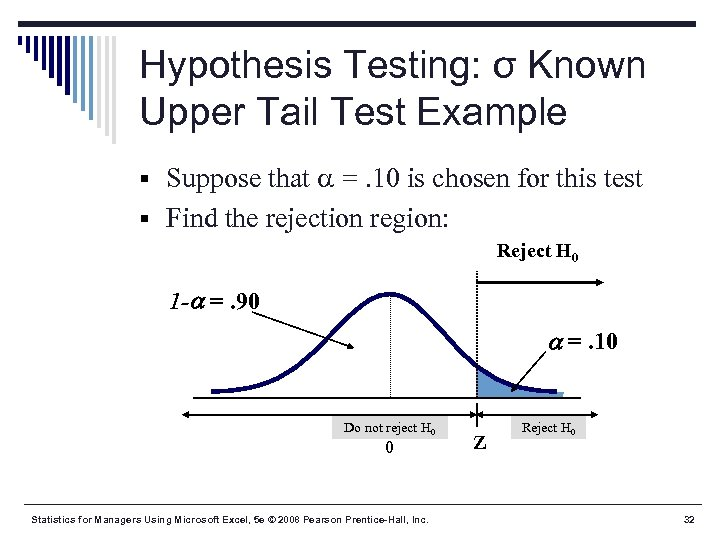Hypothesis Testing: σ Known Upper Tail Test Example § Suppose that =. 10 is chosen for this test § Find the rejection region: Reject H 0 1 - =. 90 =. 10 Do not reject H 0 0 Statistics for Managers Using Microsoft Excel, 5 e © 2008 Pearson Prentice-Hall, Inc. Z Reject H 0 32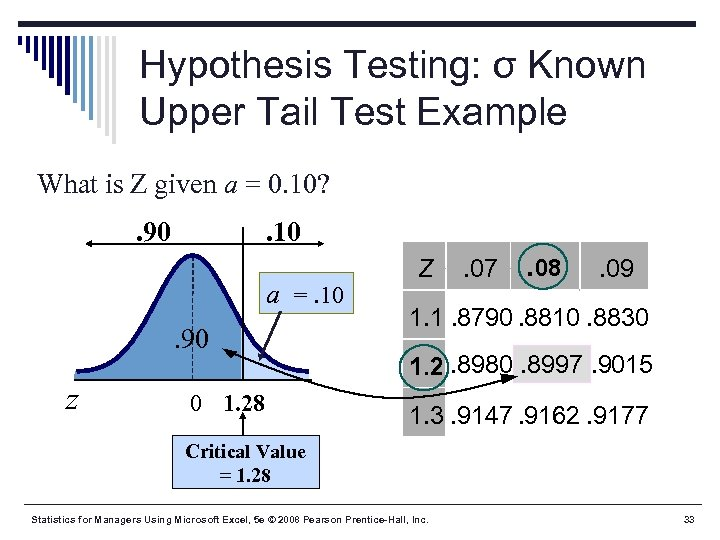Hypothesis Testing: σ Known Upper Tail Test Example What is Z given a = 0. 10? . 90 . 10 a =. 10. 90 z 0 1. 28 Z . 07 . 08 . 09 1. 1. 8790. 8810. 8830 1. 2. 8980. 8997. 9015 1. 3. 9147. 9162. 9177 Critical Value = 1. 28 Statistics for Managers Using Microsoft Excel, 5 e © 2008 Pearson Prentice-Hall, Inc. 33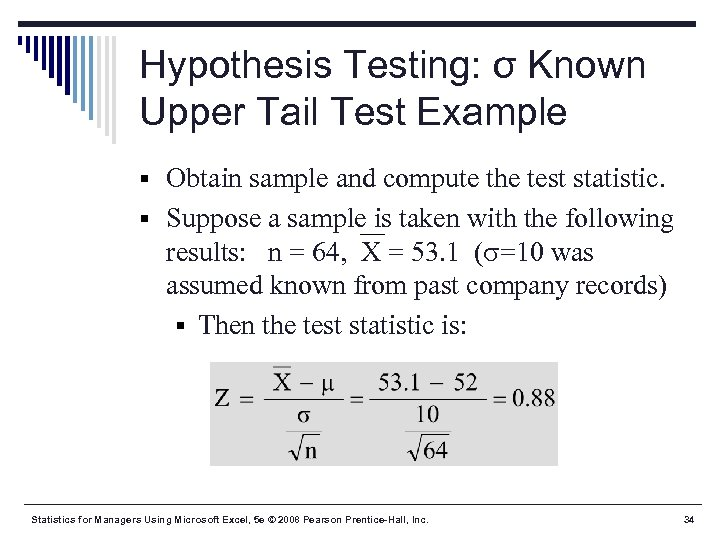Hypothesis Testing: σ Known Upper Tail Test Example § Obtain sample and compute the test statistic. § Suppose a sample is taken with the following results: n = 64, X = 53. 1 ( =10 was assumed known from past company records) § Then the test statistic is: Statistics for Managers Using Microsoft Excel, 5 e © 2008 Pearson Prentice-Hall, Inc. 34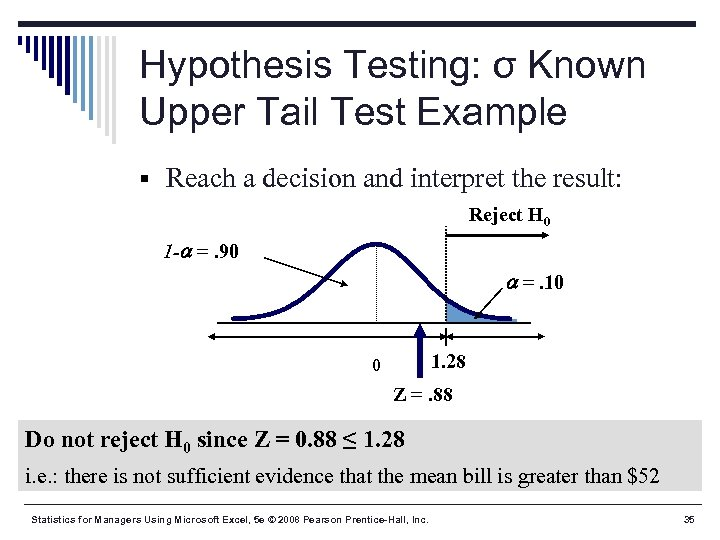Hypothesis Testing: σ Known Upper Tail Test Example § Reach a decision and interpret the result: Reject H 0 1 - =. 90 =. 10 1. 28 0 Z =. 88 Do not reject H 0 since Z = 0. 88 ≤ 1. 28 i. e. : there is not sufficient evidence that the mean bill is greater than \$52 Statistics for Managers Using Microsoft Excel, 5 e © 2008 Pearson Prentice-Hall, Inc. 35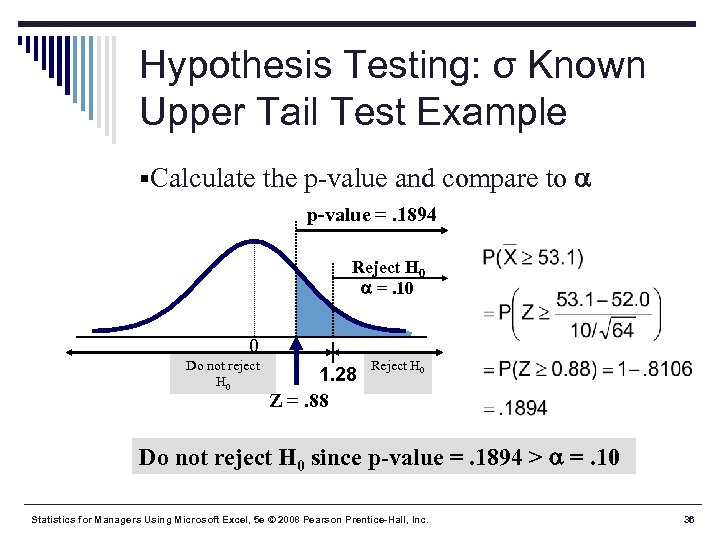Hypothesis Testing: σ Known Upper Tail Test Example §Calculate the p-value and compare to p-value =. 1894 Reject H 0 =. 10 0 Do not reject H 0 1. 28 Z =. 88 Reject H 0 Do not reject H 0 since p-value =. 1894 > =. 10 Statistics for Managers Using Microsoft Excel, 5 e © 2008 Pearson Prentice-Hall, Inc. 36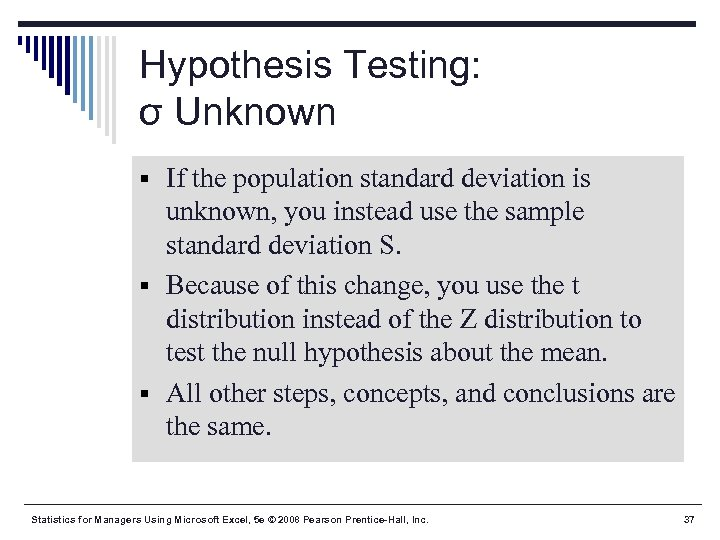Hypothesis Testing: σ Unknown § If the population standard deviation is unknown, you instead use the sample standard deviation S. § Because of this change, you use the t distribution instead of the Z distribution to test the null hypothesis about the mean. § All other steps, concepts, and conclusions are the same. Statistics for Managers Using Microsoft Excel, 5 e © 2008 Pearson Prentice-Hall, Inc. 37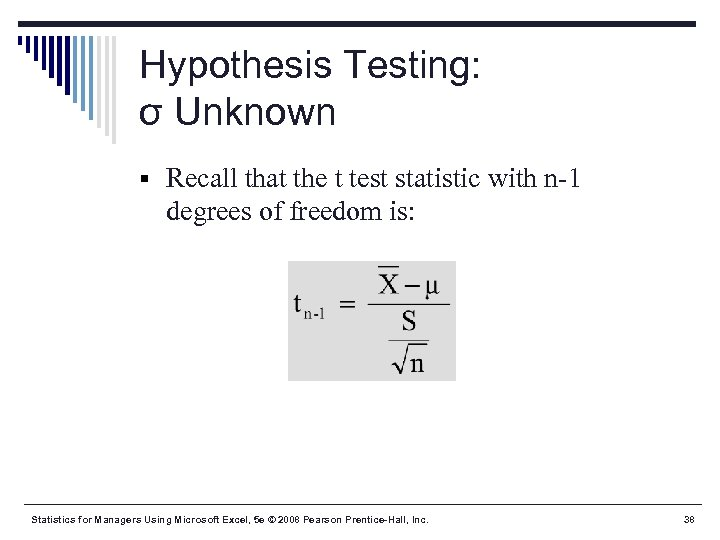Hypothesis Testing: σ Unknown § Recall that the t test statistic with n-1 degrees of freedom is: Statistics for Managers Using Microsoft Excel, 5 e © 2008 Pearson Prentice-Hall, Inc. 38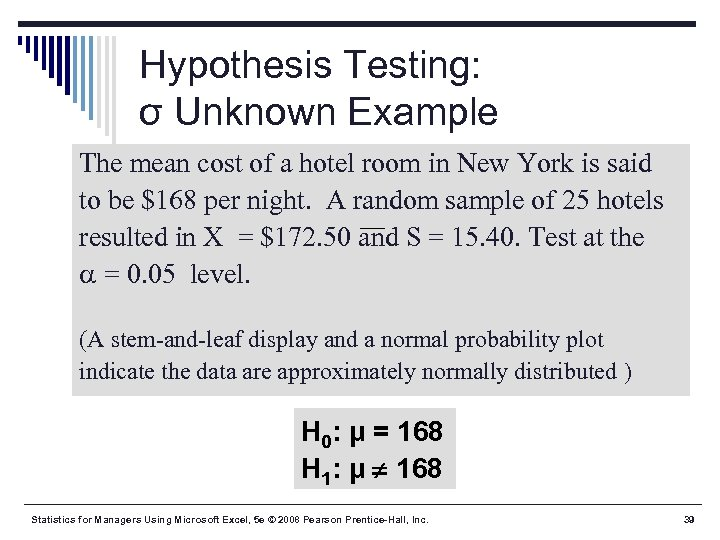Hypothesis Testing: σ Unknown Example The mean cost of a hotel room in New York is said to be \$168 per night. A random sample of 25 hotels resulted in X = \$172. 50 and S = 15. 40. Test at the = 0. 05 level. (A stem-and-leaf display and a normal probability plot indicate the data are approximately normally distributed ) H 0: μ = 168 H 1: μ ¹ 168 Statistics for Managers Using Microsoft Excel, 5 e © 2008 Pearson Prentice-Hall, Inc. 39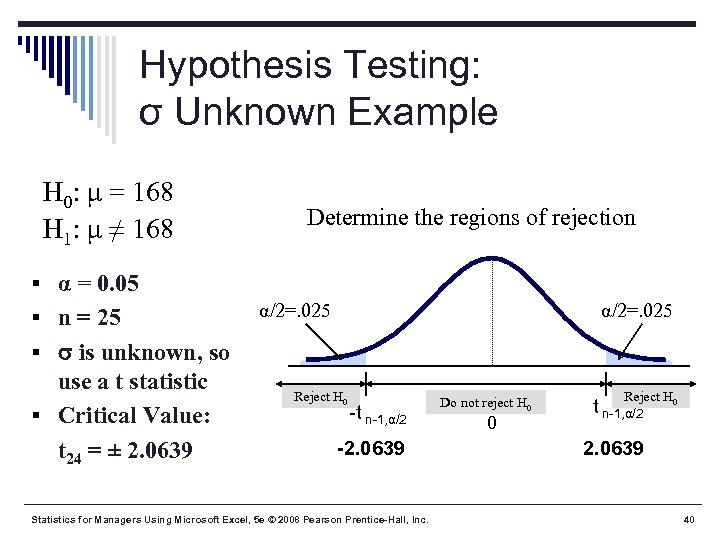Hypothesis Testing: σ Unknown Example H 0: μ = 168 H 1: μ ≠ 168 Determine the regions of rejection § α = 0. 05 § n = 25 α/2=. 025 § is unknown, so use a t statistic § Critical Value: t 24 = ± 2. 0639 Reject H 0 -t n-1, α/2 -2. 0639 Statistics for Managers Using Microsoft Excel, 5 e © 2008 Pearson Prentice-Hall, Inc. Do not reject H 0 0 Reject H 0 t n-1, α/2 2. 0639 40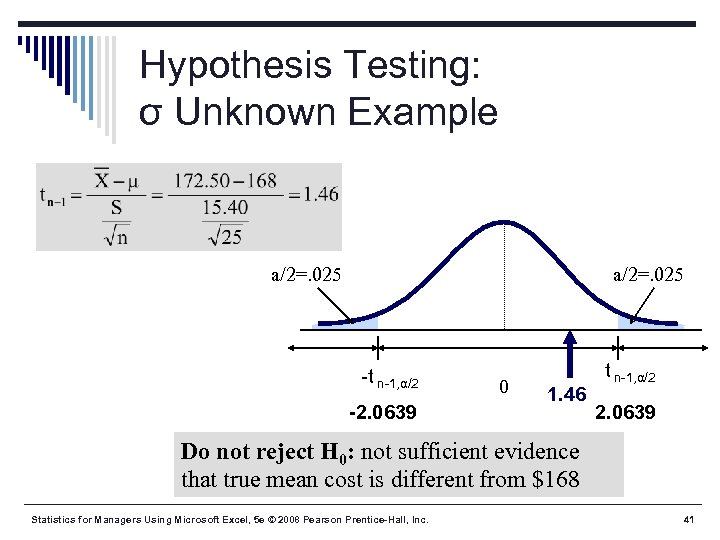Hypothesis Testing: σ Unknown Example a/2=. 025 -t n-1, α/2 -2. 0639 0 1. 46 t n-1, α/2 2. 0639 Do not reject H 0: not sufficient evidence that true mean cost is different from \$168 Statistics for Managers Using Microsoft Excel, 5 e © 2008 Pearson Prentice-Hall, Inc. 41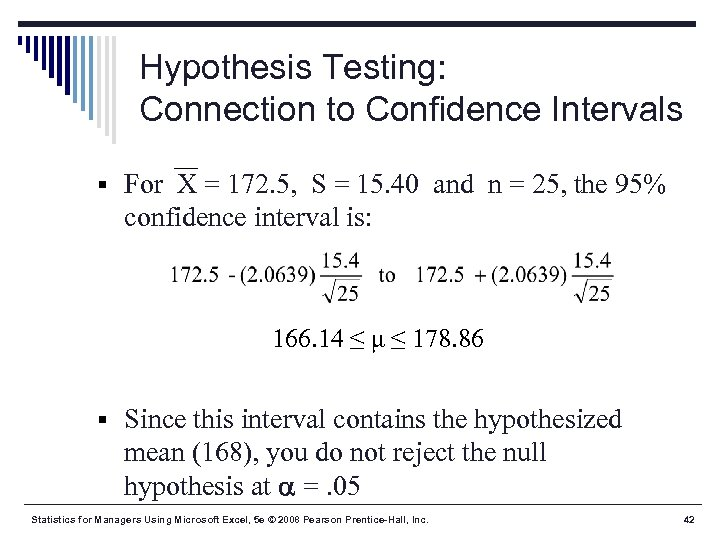Hypothesis Testing: Connection to Confidence Intervals § For X = 172. 5, S = 15. 40 and n = 25, the 95% confidence interval is: 166. 14 ≤ μ ≤ 178. 86 § Since this interval contains the hypothesized mean (168), you do not reject the null hypothesis at =. 05 Statistics for Managers Using Microsoft Excel, 5 e © 2008 Pearson Prentice-Hall, Inc. 42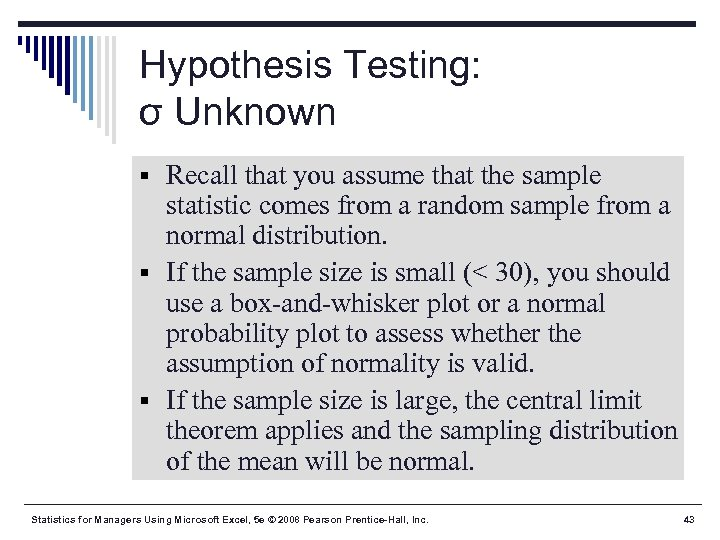Hypothesis Testing: σ Unknown § Recall that you assume that the sample statistic comes from a random sample from a normal distribution. § If the sample size is small (< 30), you should use a box-and-whisker plot or a normal probability plot to assess whether the assumption of normality is valid. § If the sample size is large, the central limit theorem applies and the sampling distribution of the mean will be normal. Statistics for Managers Using Microsoft Excel, 5 e © 2008 Pearson Prentice-Hall, Inc. 43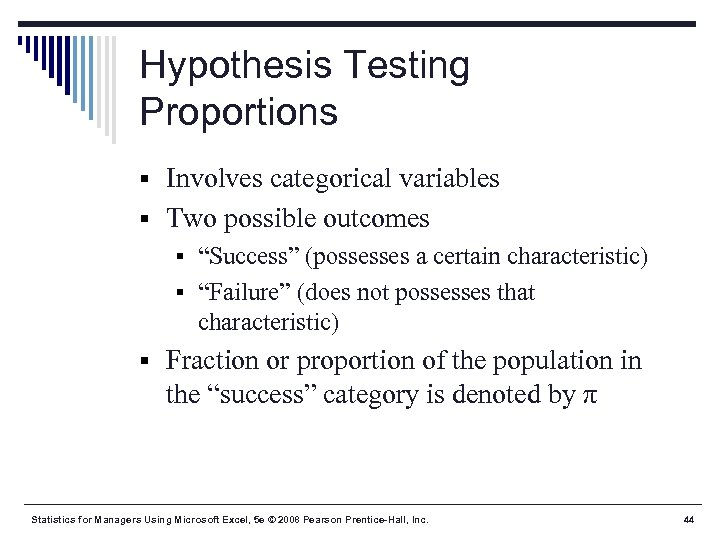Hypothesis Testing Proportions § Involves categorical variables § Two possible outcomes § “Success” (possesses a certain characteristic) § “Failure” (does not possesses that characteristic) § Fraction or proportion of the population in the “success” category is denoted by π Statistics for Managers Using Microsoft Excel, 5 e © 2008 Pearson Prentice-Hall, Inc. 44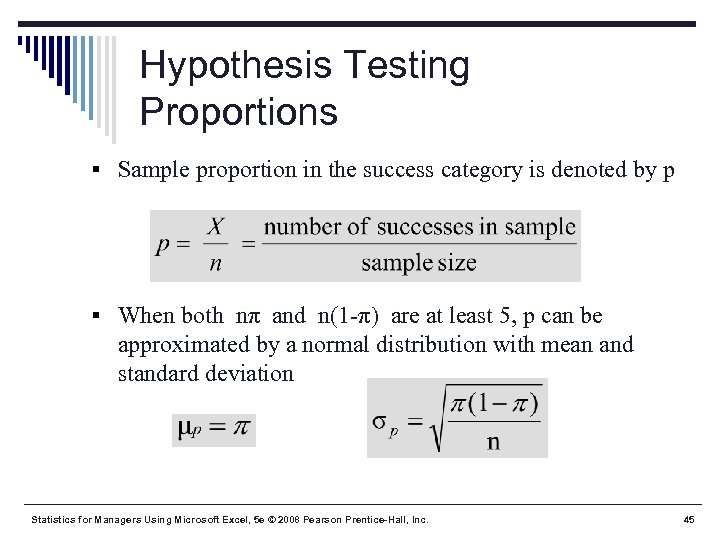Hypothesis Testing Proportions § Sample proportion in the success category is denoted by p § When both nπ and n(1 -π) are at least 5, p can be approximated by a normal distribution with mean and standard deviation Statistics for Managers Using Microsoft Excel, 5 e © 2008 Pearson Prentice-Hall, Inc. 45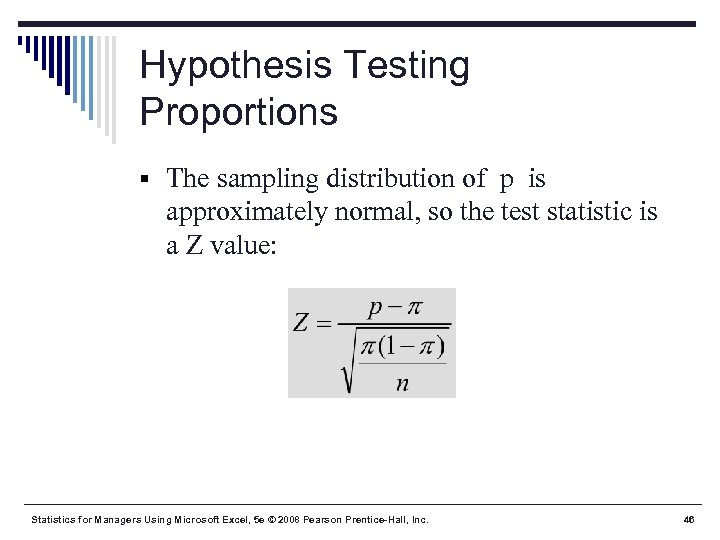Hypothesis Testing Proportions § The sampling distribution of p is approximately normal, so the test statistic is a Z value: Statistics for Managers Using Microsoft Excel, 5 e © 2008 Pearson Prentice-Hall, Inc. 46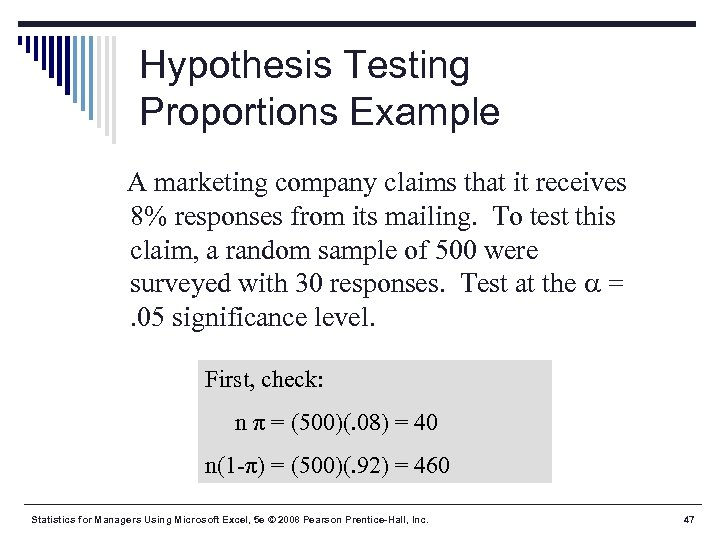Hypothesis Testing Proportions Example A marketing company claims that it receives 8% responses from its mailing. To test this claim, a random sample of 500 were surveyed with 30 responses. Test at the =. 05 significance level. First, check: n π = (500)(. 08) = 40 n(1 -π) = (500)(. 92) = 460 Statistics for Managers Using Microsoft Excel, 5 e © 2008 Pearson Prentice-Hall, Inc. 47Hypothesis Testing Proportions Example H 0: π =. 08 H 1: π ≠. 08 α =. 05 n = 500, p =. 06 Critical Values: ± 1. 96 Determine region of rejection Reject . 025 -1. 96 Statistics for Managers Using Microsoft Excel, 5 e © 2008 Pearson Prentice-Hall, Inc. 0 1. 96 z 48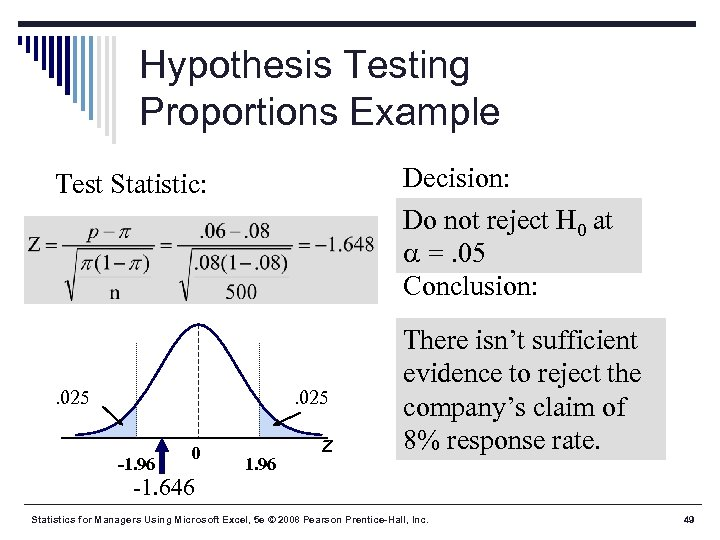Hypothesis Testing Proportions Example Decision: Do not reject H 0 at =. 05 Conclusion: Test Statistic: . 025 -1. 96 0 -1. 646 1. 96 z There isn’t sufficient evidence to reject the company’s claim of 8% response rate. Statistics for Managers Using Microsoft Excel, 5 e © 2008 Pearson Prentice-Hall, Inc. 49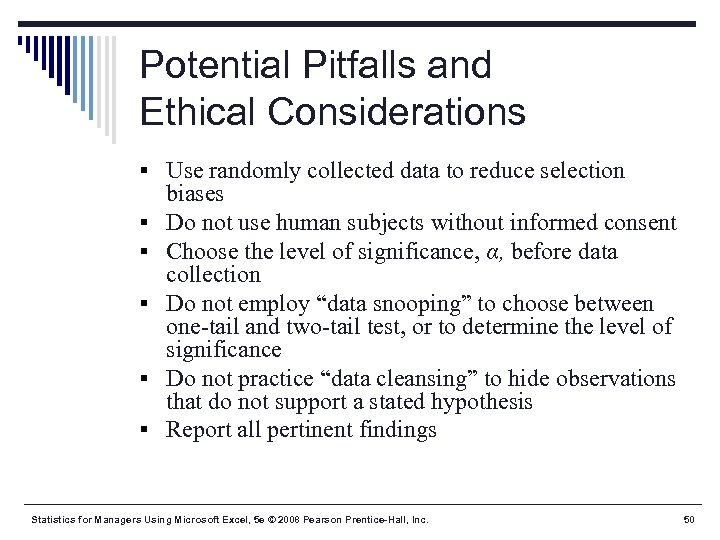Potential Pitfalls and Ethical Considerations § Use randomly collected data to reduce selection § § § biases Do not use human subjects without informed consent Choose the level of significance, α, before data collection Do not employ “data snooping” to choose between one-tail and two-tail test, or to determine the level of significance Do not practice “data cleansing” to hide observations that do not support a stated hypothesis Report all pertinent findings Statistics for Managers Using Microsoft Excel, 5 e © 2008 Pearson Prentice-Hall, Inc. 50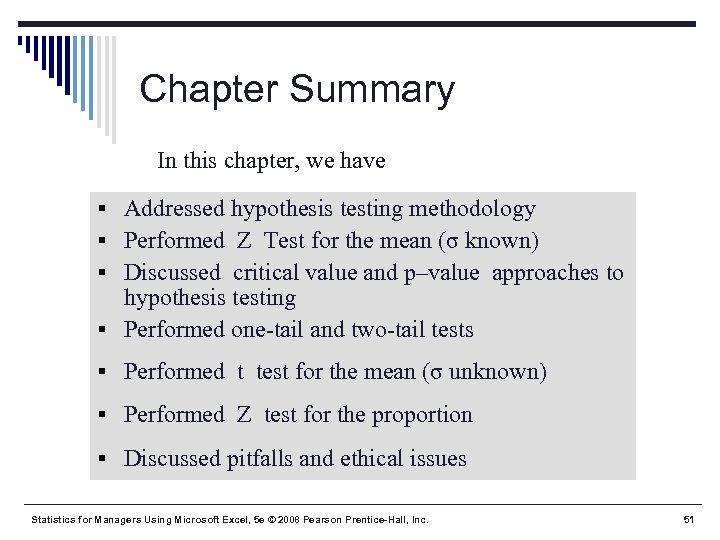Chapter Summary In this chapter, we have § Addressed hypothesis testing methodology § Performed Z Test for the mean (σ known) § Discussed critical value and p–value approaches to hypothesis testing § Performed one-tail and two-tail tests § Performed t test for the mean (σ unknown) § Performed Z test for the proportion § Discussed pitfalls and ethical issues Statistics for Managers Using Microsoft Excel, 5 e © 2008 Pearson Prentice-Hall, Inc. 51